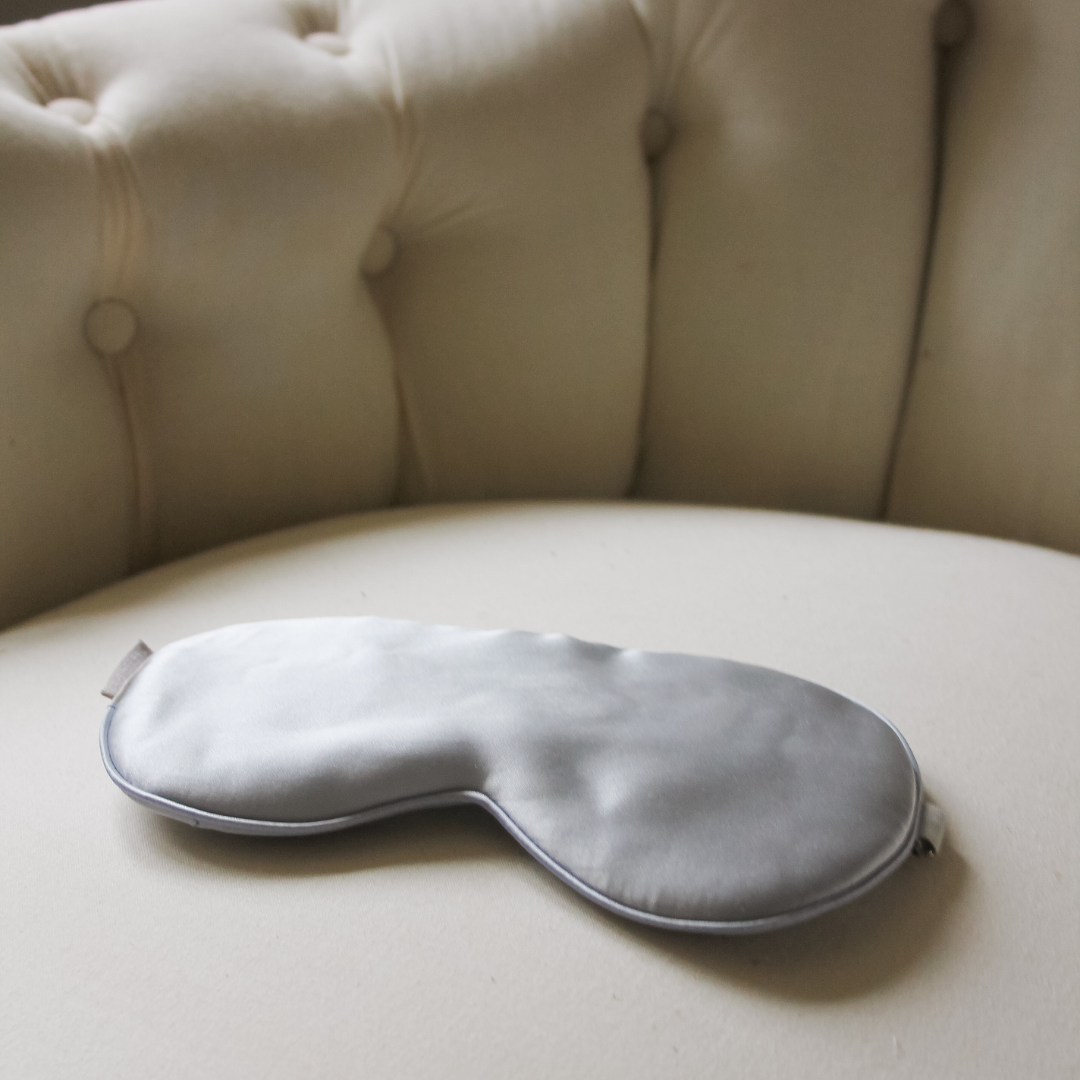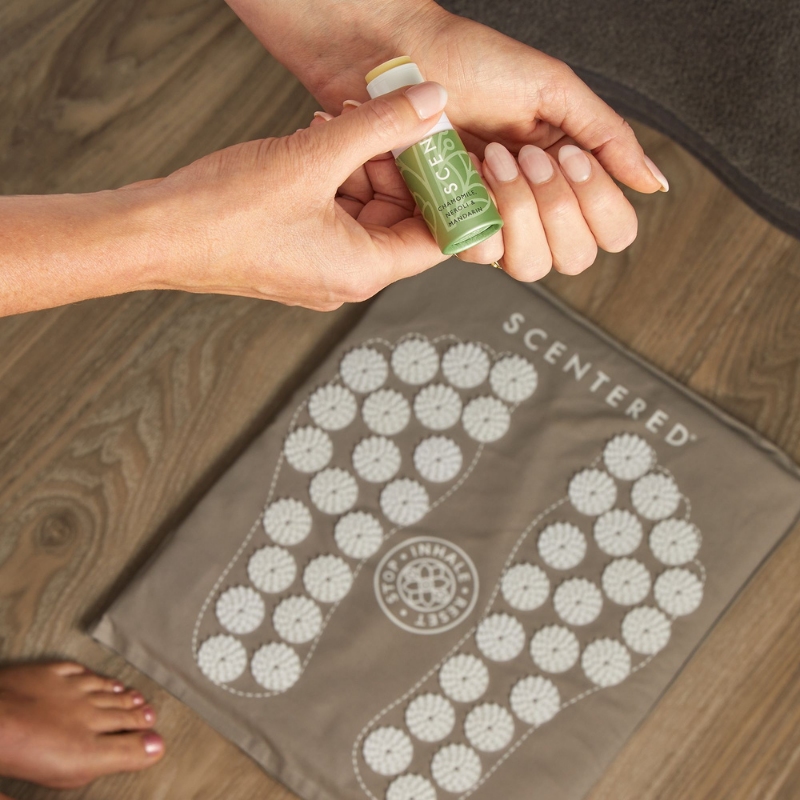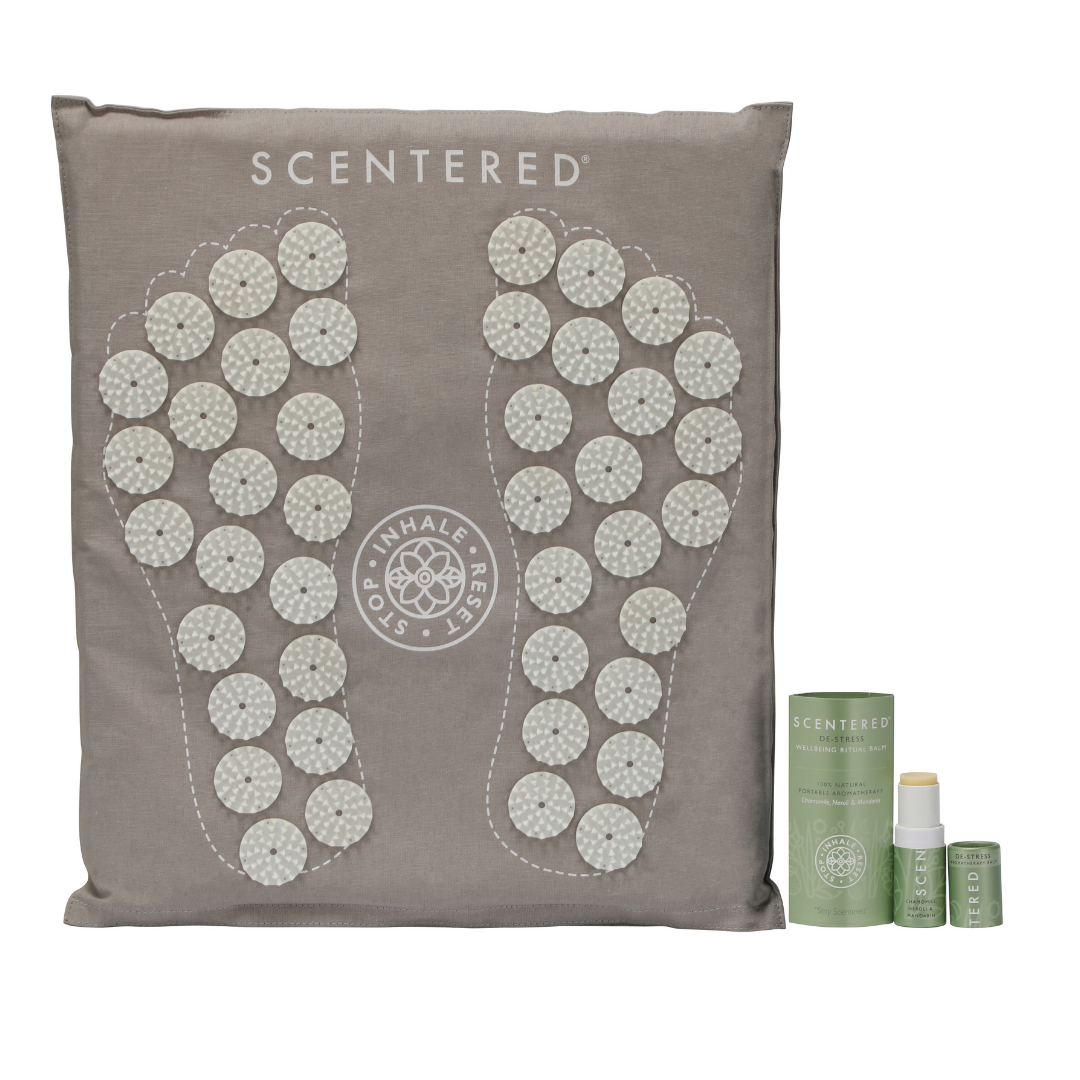# Beat Study/Work Stress

Stress can manifest itself in many ways and really affect our day to day wellbeing.  Essential oils can help with creating feelings of calm, improve concentration and aid relaxation before bedtime.### S\$i\$l\$k\$ E\$y\$

£19.99### S\$L\$E\$E\$P\$ W\$E\$L\$L\$ W\$e\$l\$l\$b\$e\$i\$n\$g\$ R\$i\$t\$

De £16.00### D\$E\$-S\$T\$R\$E\$S\$S\$ W\$e\$l\$l\$b\$e\$i\$n\$g\$ R\$i\$t\$u

De £16.00### D\$E\$-S\$T\$R\$E\$S\$S\$ H\$o\$m\$e\$ A\$r\$o\$m

De £16.95### F\$O\$C\$U\$S\$ W\$e\$l\$l\$b\$e\$i\$n\$g\$ R\$i\$t\$u\$a\$

De £16.90### C\$a\$l\$m\$ & C\$o\$n\$f\$i

£39.00### L\$u\$x\$u\$r\$y\$ W\$e\$l\$l\$b\$e\$i\$n\$g\$ S\$l\$

£29.00### De-stress Five Minute Foot Mat & Mindful Aromatherapy Balm

£35.00£29.00